Jim Dehner

Tableau How to’s,  Use Cases, and Forums Questions

Hey #DataFam

Let’s Talk

Open for

Dates are a special data type in Tableau the unique properties of being self-sequencing, sorting and can be used in date functions to set ranges, filter and aggregations.  But some legacy systems or public sources sometimes cast dates as a single string or individual strings for each date part.  Working with “real date” is much easier and converting strings to date is really not that difficult.First – how do you know if your date is really text –

Open the Data Source Tab – the data may look like a date but if the Data Type is ABC then it has been loaded as a StringThe first solution is to convert the data in the frame

Select the ABC icon and select Date for Discrete values and Date & Time for continuous valuesWhat if the date is spread over several individual fields like Month,  – January, Quarter – Q3, Period – 2019Q2  and a year that may be a number or a String.  Those cases will require a calculation before converting to a real date.

Month – year data entered as a String and Number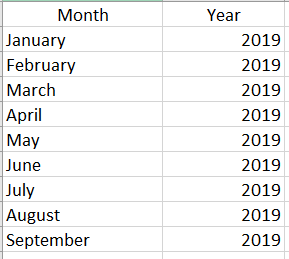Can be converted to the first of the month with a Function – Date()And it returns this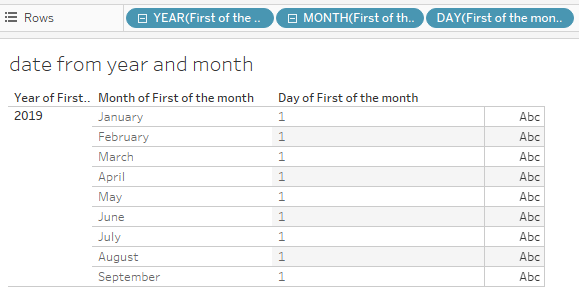Occasionally periods are entered in a string – Dateparse is a function that can be used to convert data like this: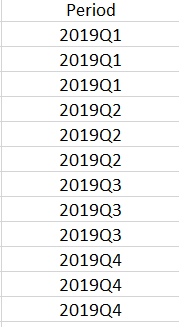The expression may look complex but if you look at it in pieces –The string in quotation just tells Tableau how the year – Month – and Date fields will be entered.

The Year is the first 4 characters of the Period field, the right 2 characters are the quarter designation – a Case statement will convert them  and the firs lot the month “01” is used for the day.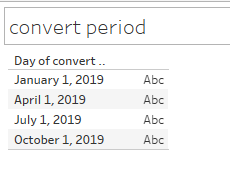One final example – converting  timestamp dates to a duration – Some users have data that includes a start and end timestamp and need to determine the duration of an event – In days, hours, minutes and seconds

The data may look like thisOne solution is to determine the duration at the lowest level – seconds and then convert the results into Days, Hours, Minutes, Seconds in separate calculations

Datediff will return the duration in seconds based on the start and end timestamps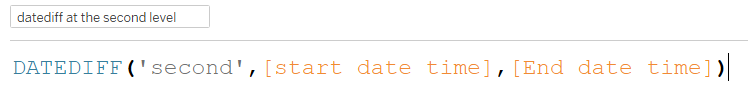Then number of days is simply the integer part of the total duration in seconds at divided by the number of seconds in a day – for example

Days =  Int([datediff at the second level]/(24*60*60))

For the remaining dateparts, make use of the Modulo function – Modulo returns the remainder of a division – e.g.   modulo will return 3 for 7/4 –

So when dividing the total seconds by the number of seconds in a day modulo will return the number of seconds left over – dividing that number by the number of seconds in an hour (3600) and taking the integer value will return the number of hours

Hours = INT(([datediff at the second level]%(24*(60)*60))/(60*60))

Minutes = INT(([datediff at the second level]%((60)*60))/(60))

Seconds = INT(([datediff at the second level]%(60)))

Finally – concatenating the parts together and adding the result to the tooltip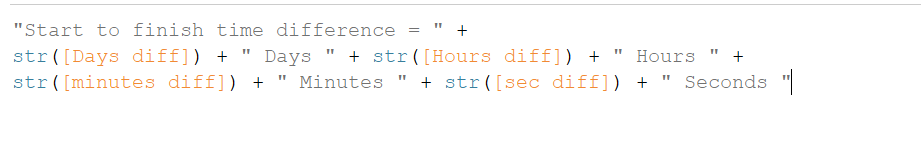Hope this helps the next time you are faced with a Date field that was entered as text.  Now practice on some data of your own

Jim